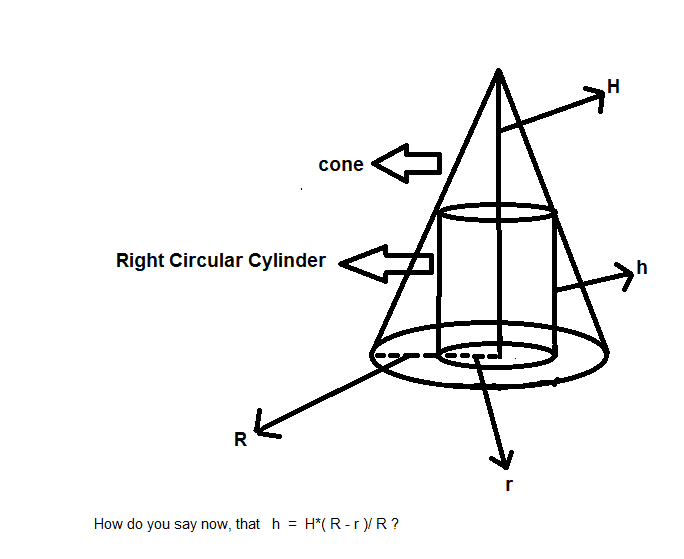# Maxima and Minima in calculus

• MHB
WMDhamnekar
MHB
Question: Prove that the radius of the right circular cylinder of greatest curved surface area which can be inscribed in a given cone is half of that of the cone.

Let r and h be the radius and height of the right circular cylinder inscribed in a given cone of radius R and height H. Let S be the curved surface area of cylinder.

S = 2πr*h

h = H*(R – r)/R
( Would any Math help board member provide me the detailed explanation of the computation of height of right circular cylinder of greatest curved surface inscribed in a given cone with a figure (as far as possible) ?

So S = 2πr*H(R – r)/R

= $\frac{2πH}{R}(r*R – r^2)$

Differentiate w.r.t.r

$\frac{dS}{dr} = \frac{2πH}{R}(R – 2r)$

For maxima or minima

$\frac{dS}{dr} =0$

=> $\frac{2πH}{R}(R – 2r) = 0$

=> R – 2r = 0

=> R = 2r

=> $r = \frac{R}{2}$

$\frac{d^2S}{dr^2} = \frac{2πH}{R}*(0 – 2)= \frac{-4πH}{R }$(negative)

So for $r = \frac{R}{2},$ S is maximum.

Kansas Boy
Draw a picture. From the side, a cone of radius R and height h looks like s triangle. I would set up a coordinate system with x-axis along the base, y-axis along the altitude, and origin at the center of the base. Then the peak is at (0, h) and one vertex is at (R, 0). The line between those two points, on the side of the cone, is given by y= -(h/R)x+ h. At x= r, y= -hr/R+ h= h(1- r/R).

The area of the curved side is $2\pi rh(1- r/R)$.

WMDhamnekar
MHB
I drew a picture describing this question. Now, how can we prove $\frac{h}{H}=\frac{(R-r)}{R}$Last edited:
Kansas Boy
The cone has height H and radius R. Set up a coordinate system so the origin is at the center of the base and the z axis passes through the vertex. Then the vertex is at (0, 0, H) and the x-axis passes through the cone at (R, 0, 0). The line through those two points, in the xz-plane, is given by $z= H\frac{R- x}{R}$.

Taking x= r, for the cylinder, we get $h= H\frac{R- r}{R}$ or, dividing both sides by H, $\frac{h}{H}= \frac{R- r}{R}$.